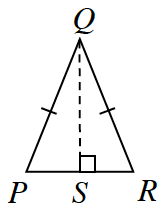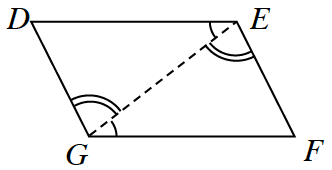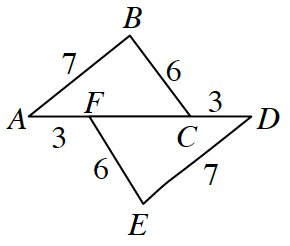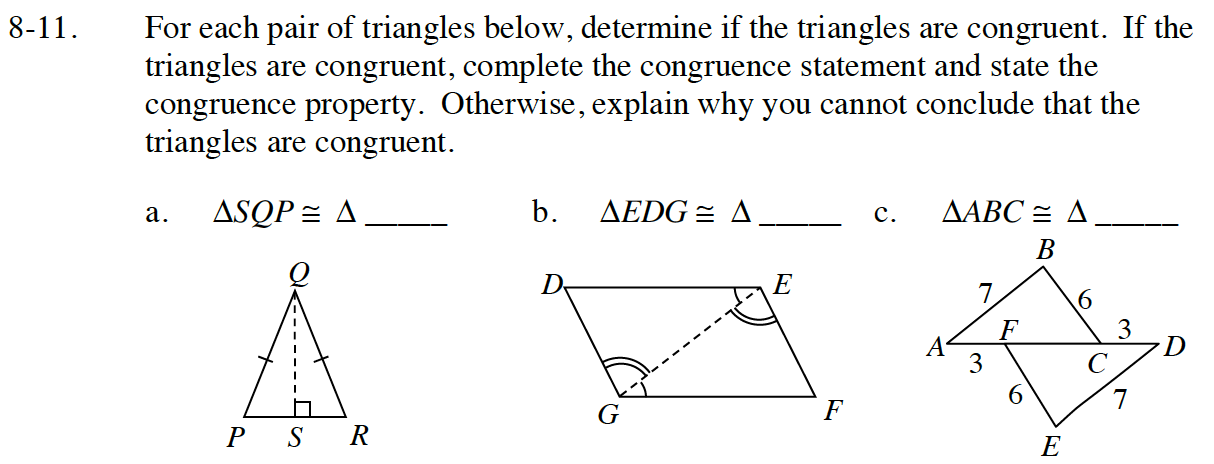### Home > INT1 > Chapter Ch8 > Lesson 8.1.1 > Problem8-11

8-11.
1. For each pair of triangles below, determine if the triangles are congruent. If the triangles are congruent, complete the congruence statement and state the congruence property. Otherwise, explain why you cannot conclude that the triangles are congruent. Homework Help ✎

2.  a. ΔSQP ≅ Δ____b. ΔEDG ≅ Δ____c. ΔABC ≅ Δ____ΔSQR; HL≅ (Remember QS = SQ, Reflexive)

ΔGFE; ASA≅ (Remember GE = EG Reflexive)

ΔDEF; SSS≅